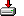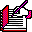#firstcap.sas```%macro FirstCap (S);

%* Richard A. DeVenezia;
%* Given an ALL caps string, convert it to one
%* where only the first letter of each word is capitalized.;

%* For use in data step only;
%* Usage:
%* %FirstCap (<name of data step variable>);
%*;

%* ensure no collision with existing dataset variables;

%local random L I c pc;
%let random = %sysfunc (ranuni(0), 10.8);
%let random = _%substr(&random,3);

%let L = L&random.L;
%let I = I&random.I;
%let c = c&random.c;
%let pc = pc&random.pc;

length &c \$1;
&L = length (&S);
&c = substr(&S,1,1);
do &I = 2 to &L;
&pc = &c;
&c = substr (&S,&I,1);
* only lowercase if current char is uppercase and the previous
* char was a letter (if no internal lowercase characters then the
* 'or' can be removed);
* add more 'or's to deal with punctuation or numbers;

if ('A' <= &c <= 'Z' and ('A' <= &pc <= 'Z' or 'a' <= &pc <= 'z')) then
substr (&S,&I,1) = lowcase(&c);
end;

drop &L &I &c &pc;
%mend FirsCap;```

Sample code

```/*
data names (keep=name);
length name \$60;
do i = 1 to 1e5;
p = 1;
name = '';

nW = 2 + 3 * ranuni (0);
do w = 1 to nW;
nL = 3 + 10 * ranuni (1);
do j = 1 to nL;
substr (name, p, 1) = byte (65 + 26 * ranuni (2));
p ++ 1;
end;
p ++ 1;
end;
output;
end;
run;

options mprint;
data names2;
set names;
newName = name;
%FirstCap (newName);
run;
*/```